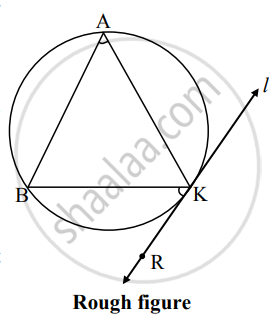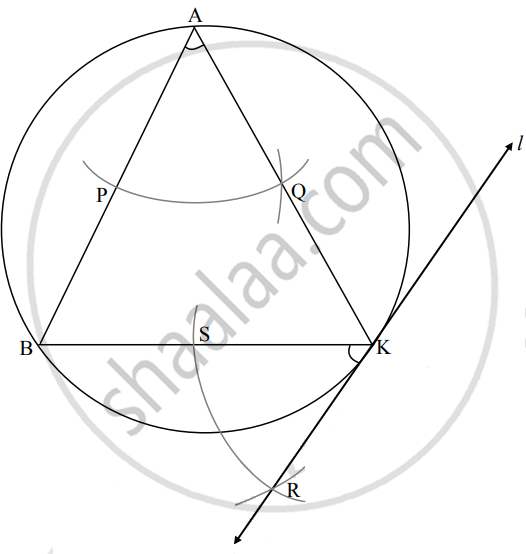# Draw a circle of radius 3 cm. Take any point K on it. Draw a tangent to the circle from point K without using center of the circle - Geometry

Diagram

Draw a circle of radius 3 cm. Take any point K on it. Draw a tangent to the circle from point K without using center of the circle.

#### Solution

Analysis:

As shown in the figure, line l is a tangent to the circle at point K.

seg BK is a chord of the circle and ∠BAK is an inscribed angle.

By tangent secant angle theorem,

∠BAK = ∠BKR

By converse of tangent secant angle theorem,

If we draw ∠BKR such that ∠BKR = ∠BAK, then ray KR

i.e. (line l) is a tangent at point K.Steps of construction:

1. Draw a circle of radius 3 cm and take any point K on it.
2. Draw chord BK of any length and an inscribed ∠BAK of any measure.
3. By taking A as a centre and any convenient distance on the compass draw an arc intersecting the arms of ∠BAK in points P and Q.
4. With K as a centre and the same distance in the compass, draw an arc intersecting the chord BK at point S.
5. Taking radius equal to PQ and S as the centre, draw an arc intersecting the previously drawn arc. Name the point of intersection as R.
6. Draw line RK. Line RK is the required tangent to the circle.
Concept: Construction of a Tangent to the Circle at a Point on the Circle
Is there an error in this question or solution?

Share